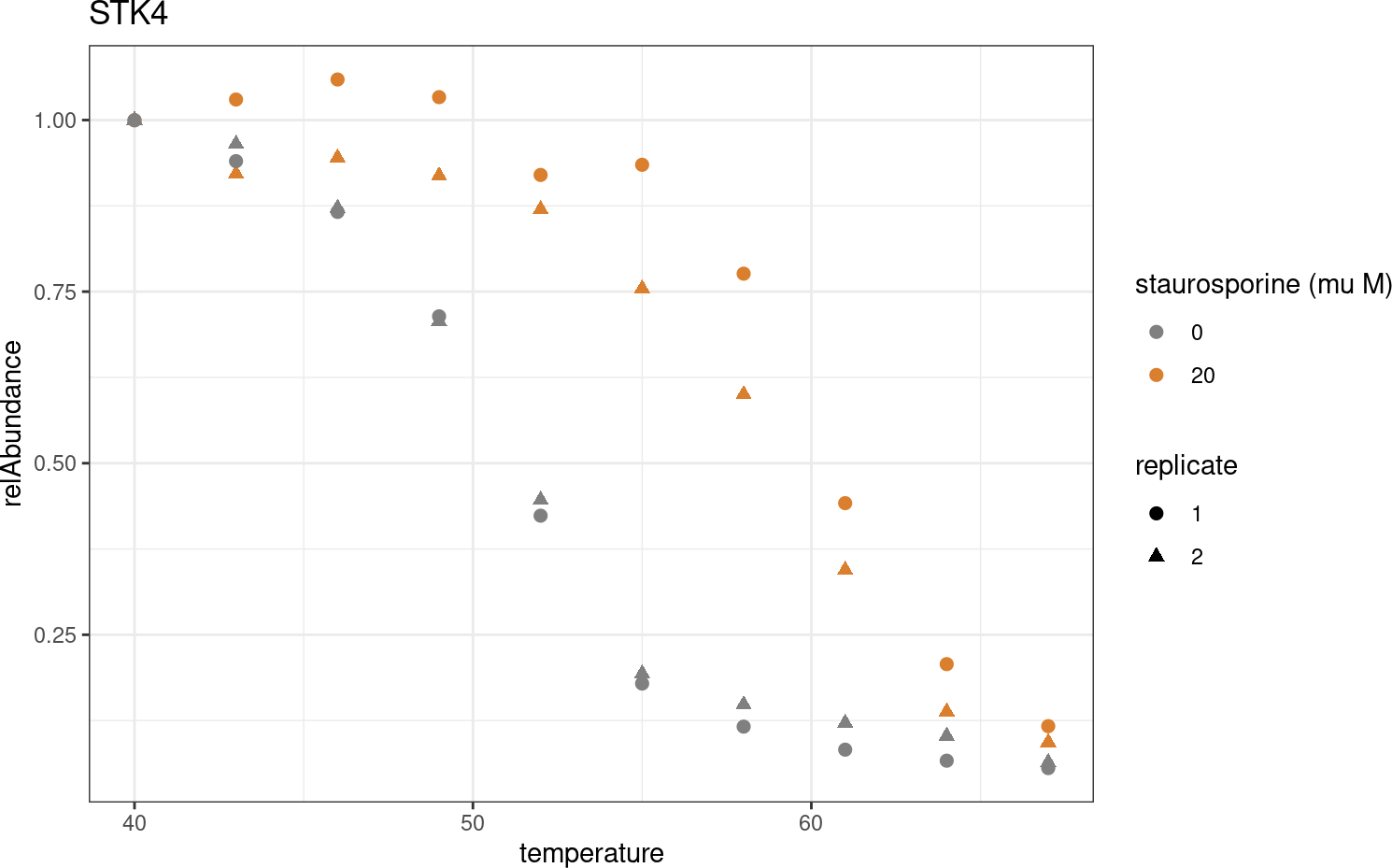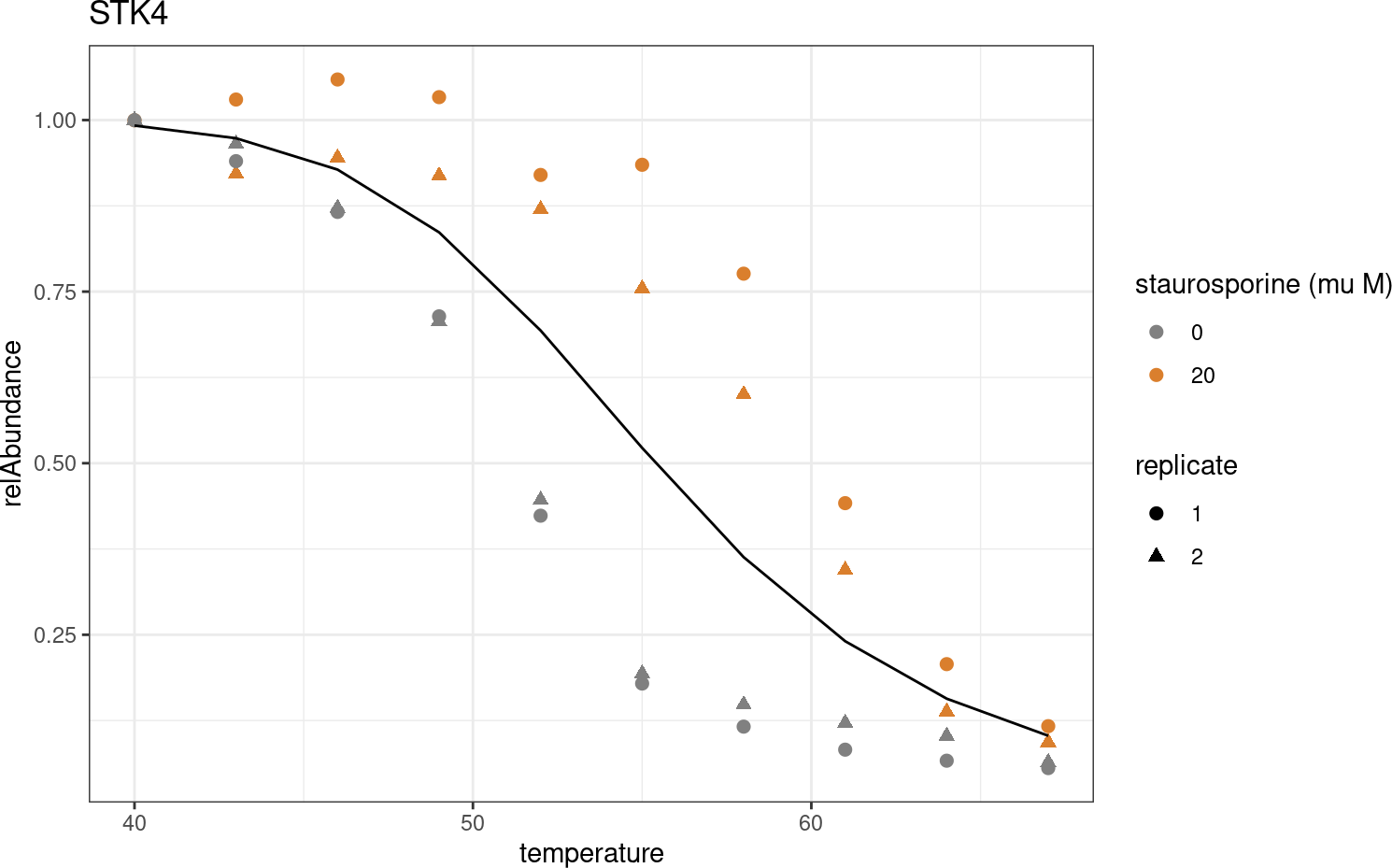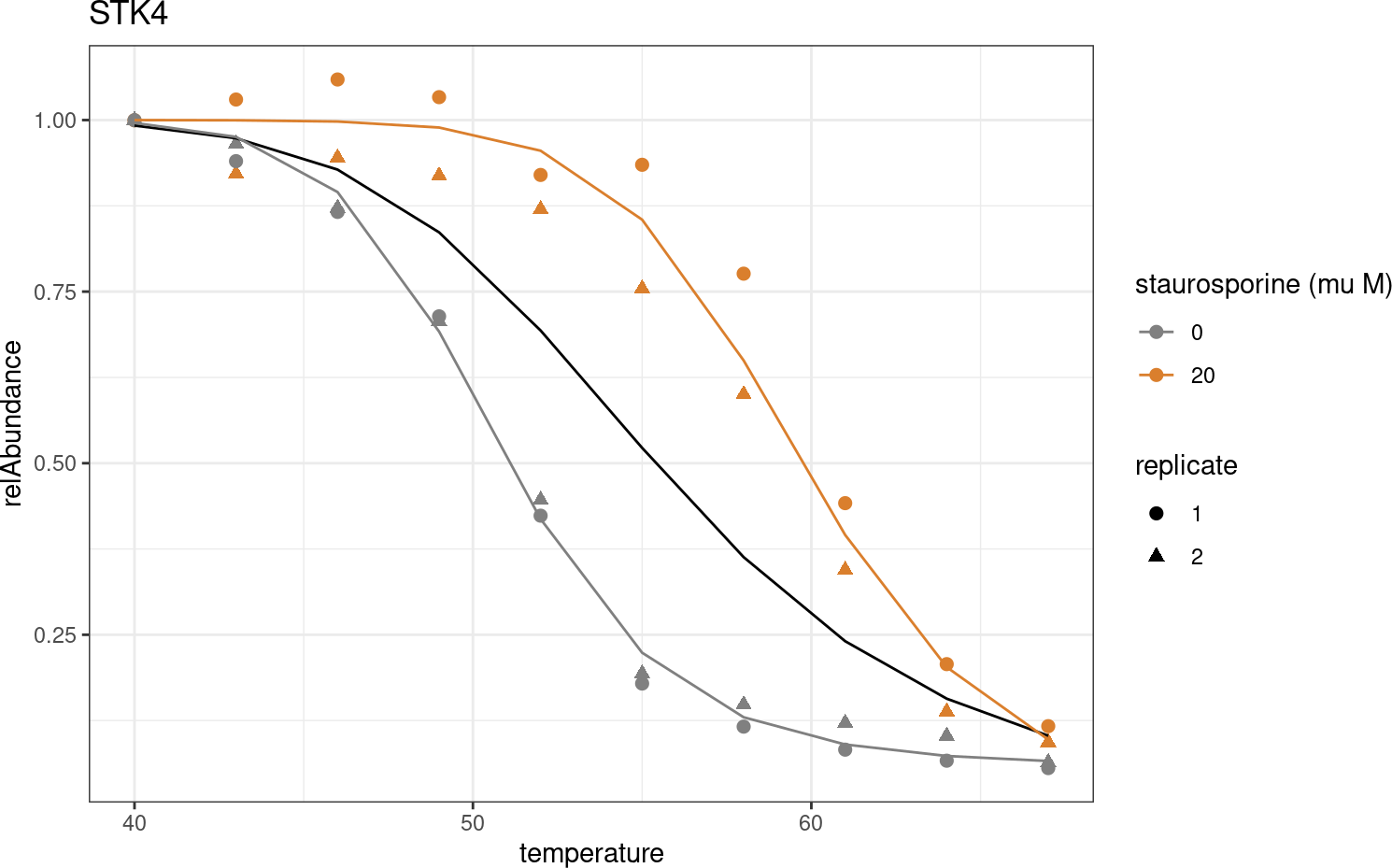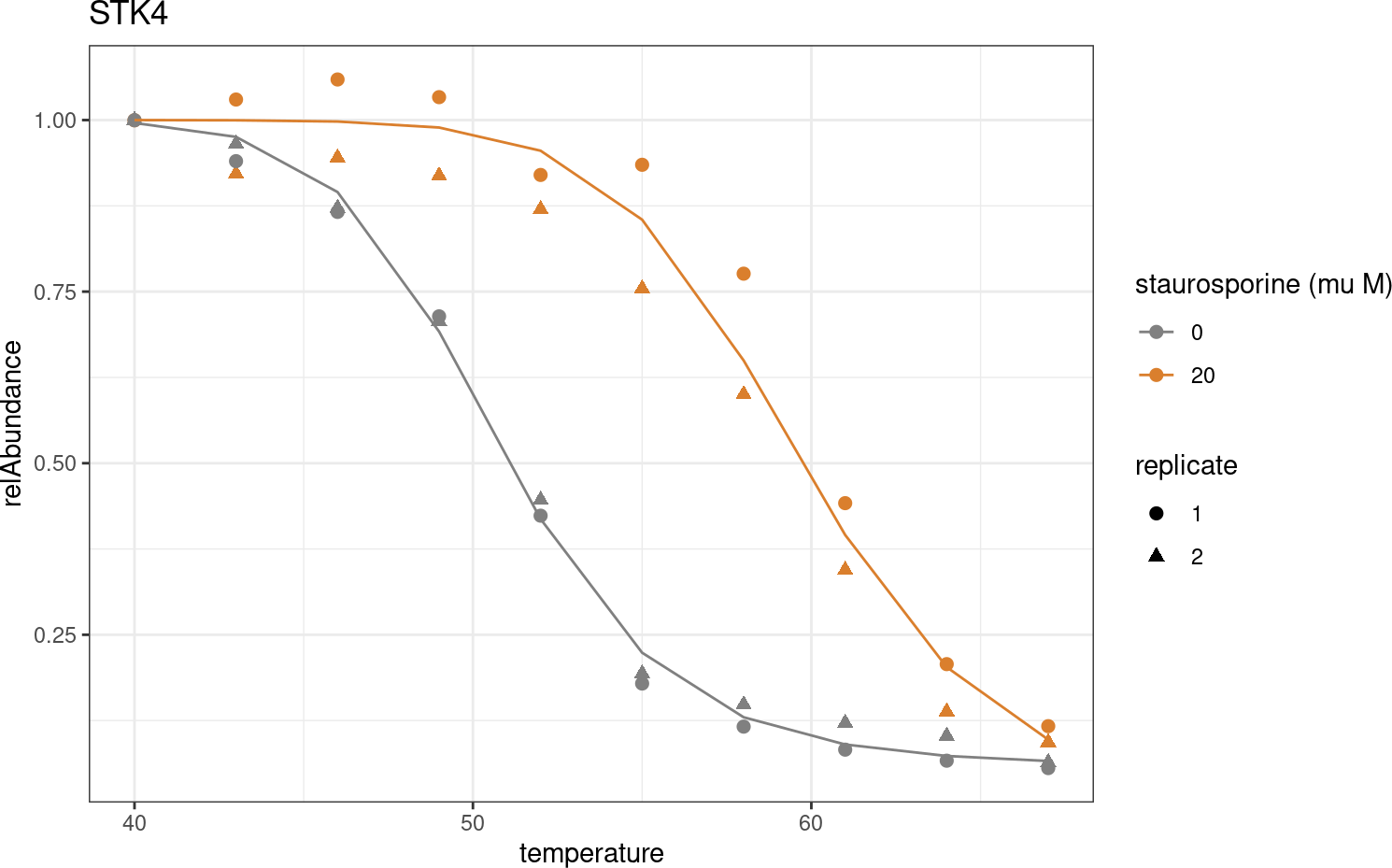# 1 Introduction

This vignette shows how to reproduce the analysis described by Childs, Bach, Franken et al. (2019): Non-parametric analysis of thermal proteome profiles reveals novel drug-binding proteins using the NPARC package.

# 2 Preparation

library(dplyr)
library(magrittr)
library(ggplot2)
library(broom)
library(knitr)
library(NPARC)

# 3 Data import

First, we load data from a staurosporine TPP experiment (Savitski et al. 2014). The necessary ETL (extract, transform, load) steps have already been conducted, including download from the supplements of the respective publication and conversion into tidy format.

data("stauro_TPP_data_tidy")

Before applying any further transformations and filters we create a copy of the imported data.

df <- stauro_TPP_data_tidy

Let’s perform a first check of the imported data:

df %>%
mutate(compoundConcentration = factor(compoundConcentration),
replicate = factor(replicate),
dataset = factor(dataset)) %>%
summary()
##           dataset         uniqueID          relAbundance     temperature
##  Staurosporine:307080   Length:307080      Min.   :  0.00   Min.   :40.0
##                         Class :character   1st Qu.:  0.17   1st Qu.:46.0
##                         Mode  :character   Median :  0.57   Median :53.5
##                                            Mean   :  0.58   Mean   :53.5
##                                            3rd Qu.:  0.95   3rd Qu.:61.0
##                                            Max.   :394.98   Max.   :67.0
##                                            NA's   :70990
##  compoundConcentration replicate  uniquePeptideMatches
##  0 :153540             1:153540   Min.   :  0.00
##  20:153540             2:153540   1st Qu.:  2.00
##                                   Median :  6.00
##                                   Mean   : 10.36
##                                   3rd Qu.: 13.00
##                                   Max.   :351.00
##                                   NA's   :63400

The displayed data contains the following columns:

• dataset: The dataset containing the measurements of several TMT-10 experiments. In each experiment, cells were treated with a vehicle or with the compound in one or two concentrations, and measured at ten different temperatures.
• uniqueID: The unique identifier for each protein. In the given dataset, it contains the gene symbol concatenated by IPI id. Example: 15 KDA PROTEIN._IPI00879051, 16 KDA PROTEIN._IPI00903282, 17 KDA PROTEIN._IPI00878120, 17 KDA PROTEIN._IPI00736161, 176 KDA PROTEIN._IPI00894060, 18 KDA PROTEIN._IPI00644570 .
• relAbundance: The relative signal intensity of the protein in each experiment, scaled to the intensity at the lowest temperature.
• temperature: The temperatures corresponding to each of the ten measurements in a TMT experiment.
• compoundConcentration The concentration of the administered compound in $$\mu M$$.
• replicate: The replicate number in each experimental group. Each pair of vehicle and treatment experiments was conducted in two replicates.
• uniquePeptideMatches: The number of unique peptides with which a protein was identified.

# 4 Data preprocessing and exploration

The imported data contains 307080 rows with entries for 7677 proteins.

First, we remove all abundances that were not found with at least one unique peptide, or for which a missing value was recorded.

df %<>% filter(uniquePeptideMatches >= 1)
df %<>% filter(!is.na(relAbundance))

Next, we ensure that the dataset only contains proteins reproducibly observed with full melting curves in both replicates and treatment groups per dataset. A full melting curve is defined by the presence of measurements at all 10 temperatures for the given experimental group.

# Count full curves per protein
df %<>%
group_by(dataset, uniqueID) %>%
mutate(n = n()) %>%
group_by(dataset) %>%
mutate(max_n = max(n)) %>%
ungroup

table(distinct(df, uniqueID, n)$n) ## ## 10 20 30 40 ## 992 809 993 4505 We see that the majority of proteins contain 40 measurements. This corresponds to two full replicate curves per experimental group. We will focus on these in the current analysis. # Filter for full curves per protein: df %<>% filter(n == max_n) %>% dplyr::select(-n, -max_n) The final data contains 180200 rows with entries for 4505 proteins. This number coincides with the value reported in Table 1 of the corresponding publication (Childs et al. 2019). # 5 Illustrative example We first illustrate the principles of nonparametric analysis of response curves (NPARC) on an example protein (STK4) from the staurosporine dataset. The same protein is shown in Figures 1 and 2 of the paper. ## 5.1 Select data We first select all entries belonging to the desired protein and dataset: stk4 <- filter(df, uniqueID == "STK4_IPI00011488") The table stk4 has 40 rows with measurements of four experimental groups. They consist of two treatment groups (vehicle: $$0~\mu M$$ staurosporine, treatment: $$20~\mu M$$ staurosporine) with two replicates each. Let us look at the treatment group of replicate 1 for an example: stk4 %>% filter(compoundConcentration == 20, replicate == 1) %>% dplyr::select(-dataset) %>% kable(digits = 2) uniqueID relAbundance temperature compoundConcentration replicate uniquePeptideMatches STK4_IPI00011488 1.00 40 20 1 8 STK4_IPI00011488 1.03 43 20 1 8 STK4_IPI00011488 1.06 46 20 1 8 STK4_IPI00011488 1.03 49 20 1 8 STK4_IPI00011488 0.92 52 20 1 8 STK4_IPI00011488 0.93 55 20 1 8 STK4_IPI00011488 0.78 58 20 1 8 STK4_IPI00011488 0.44 61 20 1 8 STK4_IPI00011488 0.21 64 20 1 8 STK4_IPI00011488 0.12 67 20 1 8 To obtain a first impression of the measurements in each experimental group, we generate a plot of the measurements: stk4_plot_orig <- ggplot(stk4, aes(x = temperature, y = relAbundance)) + geom_point(aes(shape = factor(replicate), color = factor(compoundConcentration)), size = 2) + theme_bw() + ggtitle("STK4") + scale_color_manual("staurosporine (mu M)", values = c("#808080", "#da7f2d")) + scale_shape_manual("replicate", values = c(19, 17)) print(stk4_plot_orig)We will show how to add the fitted curves to this plot in the following steps. ## 5.2 Define function for model fitting To assess whether there is a significant difference between both treatment groups, we will fit a null model and an alternative models to the data. The null model fits a sigmoid melting curve through all data points irrespective of experimental condition. The alternative model fits separate melting curves per experimental group . ## 5.3 Fit and plot null models We use the NPARC package function fitSingleSigmoid to fit the null model: nullFit <- NPARC:::fitSingleSigmoid(x = stk4$temperature, y = stk4$relAbundance) The function returns an object of class nls: summary(nullFit) ## ## Formula: y ~ (1 - Pl)/(1 + exp((b - a/x))) + Pl ## ## Parameters: ## Estimate Std. Error t value Pr(>|t|) ## Pl 0.0000 0.1795 0.000 1.00000 ## a 692.6739 226.9106 3.053 0.00419 ** ## b 12.5048 4.4989 2.780 0.00851 ** ## --- ## Signif. codes: 0 '***' 0.001 '**' 0.01 '*' 0.05 '.' 0.1 ' ' 1 ## ## Residual standard error: 0.1814 on 37 degrees of freedom ## ## Algorithm "port", convergence message: relative convergence (4) The function augment from the broom package provides a convenient way to obtain the predictions and residuals at each temperature in tabular format. By appending the returned predictions and residuals to our measurements, we ensure that relevant data is collected in the same table and can be added to the plot for visualization. The residuals will be needed later for construction of the test statistic: nullPredictions <- broom::augment(nullFit) Let us look at the values returned by augment at two consecutive temperatures. Note that, while the predictions will be the same for each experiment at a given temperature, the residuals will differ because they were computed by comparing the predictions to the actual measurements: nullPredictions %>% filter(x %in% c(46, 49)) %>% kable() x y .fitted .resid 46 1.0591140 0.9278000 0.1313139 49 1.0333794 0.8363683 0.1970111 46 0.9449568 0.9278000 0.0171568 49 0.9187253 0.8363683 0.0823571 46 0.8661451 0.9278000 -0.0616550 49 0.7139894 0.8363683 -0.1223788 46 0.8717407 0.9278000 -0.0560594 49 0.7068211 0.8363683 -0.1295471 Now we can append these values to our data frame and show the predicted curve in the plot: stk4$nullPrediction <- nullPredictions$.fitted stk4$nullResiduals <- nullPredictions$.resid stk4_plot <- stk4_plot_orig + geom_line(data = stk4, aes(y = nullPrediction)) print(stk4_plot)## 5.4 Fit and plot alternative models Next we fit the alternative model. Again, we compute the predicted values and the corresponding residuals by the broom::augment() function. To take the compound concentration as a factor into account, we iterate over both concentrations and fit separate models to each subset. We implement this by first grouping the data using the function dplyr::group_by(), and starting the model fitting by dplyr::do(). alternativePredictions <- stk4 %>% # Fit separate curves per treatment group: group_by(compoundConcentration) %>% do({ fit = NPARC:::fitSingleSigmoid(x = .$temperature, y = .$relAbundance, start=c(Pl = 0, a = 550, b = 10)) broom::augment(fit) }) %>% ungroup %>% # Rename columns for merge to data frame: dplyr::rename(alternativePrediction = .fitted, alternativeResiduals = .resid, temperature = x, relAbundance = y) Add the predicted values and corresponding residuals to our data frame: stk4 <- stk4 %>% left_join(alternativePredictions, by = c("relAbundance", "temperature", "compoundConcentration")) %>% distinct() Add the curves predicted by the alternative model to the plot. Conceptually, it corresponds to the plot shown in Figures 2 (A)/(B) of the paper. stk4_plot <- stk4_plot + geom_line(data = distinct(stk4, temperature, compoundConcentration, alternativePrediction), aes(y = alternativePrediction, color = factor(compoundConcentration))) print(stk4_plot)This plot summarizes Figures 2(A) and 2(B) in the corresponding publication (Childs et al. 2019). ## 5.5 Compute RSS values In order to quantify the improvement in goodness-of-fit of the alternative model relative to the null model, we compute the sum of squared residuals (RSS): rssPerModel <- stk4 %>% summarise(rssNull = sum(nullResiduals^2), rssAlternative = sum(alternativeResiduals^2)) kable(rssPerModel, digits = 4) rssNull rssAlternative 1.2181 0.0831 These values will be used to construct the $$F$$-statistic according to $\begin{equation} \label{eq:f_stat} {F} = \frac{{d}_{2}}{{d}_{1}} \cdot \frac{{RSS}^{0} - {RSS}^{1}}{{RSS}^{1}}. \end{equation}$ To compute this statistic and to derive a p-value, we need the degrees of freedom $${d}_{1}$$ and $${d}_{2}$$. As described in the paper, they cannot be analytically derived due to the correlated nature of the measurements. The paper describes how to estimate these values from the RSS-values of all proteins in the dataset. In the following Section, we illustrate how to repeat the model fitting for all proteins of a dataset and how to perform hypothesis testing on these models. # 6 Extend the analysis to all proteins This section describes the different steps of the NPARC workflow for model fitting and hyothesis testing. Note that the package also provides a function runNPARC() that performs all of the following steps with one single function call. ## 6.1 Start fitting In order to analyze all datasets as described in the paper, we fit null and alternative models to all proteins using the package function NPARCfit: BPPARAM <- BiocParallel::SerialParam(progressbar = FALSE) fits <- NPARCfit(x = df$temperature,
y = df$relAbundance, id = df$uniqueID,
groupsNull = NULL,
groupsAlt = df$compoundConcentration, BPPARAM = BPPARAM, returnModels = FALSE) ## Starting model fitting... ## ... complete ## Elapsed time: 48.72 secs ## Flagging successful model fits... ## ... complete. ## Evaluating model fits... ## Evaluating models ... ## ... complete. ## Computing model predictions and residuals ... ## ... complete. ## Starting model fitting... ## ... complete ## Elapsed time: 1.62 mins ## Flagging successful model fits... ## ... complete. ## Evaluating model fits... ## Evaluating models ... ## ... complete. ## Computing model predictions and residuals ... ## ... complete. str(fits, 1) ## List of 2 ##$ predictions: tibble [360,094 × 7] (S3: tbl_df/tbl/data.frame)
##  $metrics : tibble [13,515 × 15] (S3: tbl_df/tbl/data.frame) The returned object fits contains two tables. The table metrics contains the fitted parameters and goodness-of-fit measures for the null and alternative models per protein and group. The table predictions contains the corresponding predicted values and residuals per model. fits$metrics %>%
mutate(modelType = factor(modelType), nCoeffs = factor(nCoeffs), nFitted = factor(nFitted), group = factor((group))) %>%
summary
##        modelType         id                  tm                 a
##  alternative:9010   Length:13515       Min.   :      44   Min.   :   51.66
##  null       :4505   Class :character   1st Qu.:      50   1st Qu.:  837.27
##                     Mode  :character   Median :      53   Median : 1103.07
##                                        Mean   :    6977   Mean   : 1433.96
##                                        3rd Qu.:      56   3rd Qu.: 1489.30
##                                        Max.   :88122289   Max.   :15000.00
##                                        NA's   :786        NA's   :14
##        b                   pl               aumc          resid_sd
##  Min.   :  0.00001   Min.   :0.00000   Min.   : 4.87   Min.   :0.007522
##  1st Qu.: 16.12196   1st Qu.:0.05277   1st Qu.:11.56   1st Qu.:0.035041
##  Median : 21.44344   Median :0.07971   Median :14.46   Median :0.049798
##  Mean   : 27.05821   Mean   :0.14058   Mean   :15.03   Mean   :0.064141
##  3rd Qu.: 28.40230   3rd Qu.:0.14426   3rd Qu.:18.06   3rd Qu.:0.074660
##  Max.   :250.00000   Max.   :1.50000   Max.   :37.42   Max.   :1.896479
##  NA's   :14          NA's   :14        NA's   :14      NA's   :14
##  Min.   : 0.00113   Min.   :-70.47   Min.   :0.000e+00   3   :13501
##  1st Qu.: 0.02922   1st Qu.: 27.31   1st Qu.:0.000e+00   NA's:   14
##  Median : 0.06361   Median : 37.21   Median :0.000e+00
##  Mean   : 0.19878   Mean   : 40.00   Mean   :2.785e+12
##  3rd Qu.: 0.15090   3rd Qu.: 49.53   3rd Qu.:1.000e+00
##  Max.   :79.39804   Max.   :123.31   Max.   :9.691e+15
##  NA's   :14         NA's   :14       NA's   :789
##  nFitted        conv          group
##  20  :8998   Mode :logical   0   :4505
##  40  :4503   FALSE:14        20  :4505
##  NA's:  14   TRUE :13501     NA's:4505
##
##
##
## 
fits$predictions %>% mutate(modelType = factor(modelType), group = factor((group))) %>% summary ## modelType id x y ## alternative:179972 Length:360094 Min. :40.0 Min. :0.0000 ## null :180122 Class :character 1st Qu.:46.0 1st Qu.:0.1563 ## Mode :character Median :53.5 Median :0.5782 ## Mean :53.5 Mean :0.5643 ## 3rd Qu.:61.0 3rd Qu.:0.9570 ## Max. :67.0 Max. :8.2379 ## NA's :14 NA's :14 ## .fitted .resid group ## Min. :0.01099 Min. :-1.156751 0 : 89967 ## 1st Qu.:0.15960 1st Qu.:-0.025273 20 : 90005 ## Median :0.58435 Median : 0.000311 NA's:180122 ## Mean :0.55996 Mean : 0.004377 ## 3rd Qu.:0.96268 3rd Qu.: 0.028117 ## Max. :1.50000 Max. : 7.240277 ## NA's :14 NA's :14 ## 6.2 Check example The results of the STK4 example from earlier can be selected from this object as follows. First, we check the RSS values of the null and alterantive models: stk4Metrics <- filter(fits$metrics, id == "STK4_IPI00011488")

rssNull <- filter(stk4Metrics, modelType == "null")$rss rssAlt <- sum(filter(stk4Metrics, modelType == "alternative")$rss) # Summarize over both experimental groups

rssNull
##  1.218132
rssAlt
##  0.08314745

Next, we plot the predicted curves per model and experimental group:

stk4Predictions <- filter(fits$predictions, modelType == "alternative", id == "STK4_IPI00011488") stk4_plot_orig + geom_line(data = filter(stk4Predictions, modelType == "alternative"), aes(x = x, y = .fitted, color = factor(group))) + geom_line(data = filter(stk4Predictions, modelType == "null"), aes(x = x, y = .fitted))# 7 Compute test statistics ## 7.1 Why we need to estimate the degrees of freedom In order to compute $$F$$-statistics per protein and dataset according to Equation (), we need to know the degrees of freedom of the corresponding null distribution. If we could assume independent and identically distributed (iid) residuals, we could compute them from the number of fitted values and model parameters. In the following, we will show why this simple equation is not appropriate for the curve data we are working with. First, we compute the test statistics and p-values with theoretical degrees of freedom. These would be true for the case of iid residuals: modelMetrics <- fits$metrics
fStats <- NPARCtest(modelMetrics, dfType = "theoretical")

Let us take a look at the computed degrees of freedom:

fStats %>%
distinct(nFittedNull, nFittedAlt, nCoeffsNull, nCoeffsAlt, df1, df2) %>%
kable()
df1 df2 nFittedNull nFittedAlt nCoeffsNull nCoeffsAlt
3 34 40 40 3 6

We plot the $$F$$-statistics against the theoretical $$F$$-distribution to check how well the null distribution is approximated now:

ggplot(filter(fStats, !is.na(pAdj))) +
geom_density(aes(x = fStat), fill = "steelblue", alpha = 0.5) +
geom_line(aes(x = fStat, y = df(fStat, df1 = df1, df2 = df2)), color = "darkred", size = 1.5) +
theme_bw() +
# Zoom in to small values to increase resolution for the proteins under H0:
xlim(c(0, 10))
## Warning: Removed 178 rows containing non-finite values (stat_density).
## Warning: Removed 178 row(s) containing missing values (geom_path).